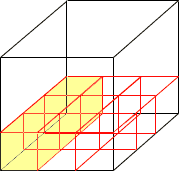to start this seems like a good place for students to learn. and probably shouldn't bother you seeing i am not in school, am an old man 51, if you have the time this is a concrete problem. seeing they sell concrete by cubic yards. if you have a 10" dia pipe 8' long how many cubic yards would it take to fill with concrete. how how would you go about figuring it out. and also if you would how many cubic feet, cubic inches in a cubic yard. i know you don't need to answer, seeing i am not in school, but would appreaciate it. michael, out of school, design machines and other things. Hi Michael, Since you want the volume in cubic yards I would put all the measurements in yards. There are 36" in a yard and hence 10" is  10/36 = 0.2778 yards There are 3 feet in a yard so 8' is  8/3 = 2.667 yards The volume of a cylinder is pi r 2 h where r is the radius, h is the height and pi is approximately 3.1416. The volume of your pipe is then 3.1416 x ( 0.2778/2) 2 x 2.667 = 0.16 cubic yards Now suppose that you have a box that is 1yard by 1 yard by 1 yard, that is 1 cubic yard. Imagine also that you have a collection of blocks that are 1 foot by 1 foot by 1 foot, that is each is 1 cubic foot. How many 1 cubic foot blocks can you fit in 1 cubic yard? I started to fill the box in the diagram below.Since thet are 3 feet in a yard, the bottom layer is 3 x 3 = 9 blocks. It takes three layers to fill the box and hence there are 3 x 3 x 3 = 27 cubic feet in a cubic yard. If you want to fill the 1 cubic yard box with blocks that are 1 in by 1 inch by 1 inch use the same procedure. You get 36 x 36 x 36 = 46656 cubic inches in a cubic yard. Cheers, Penny Go to Math Central